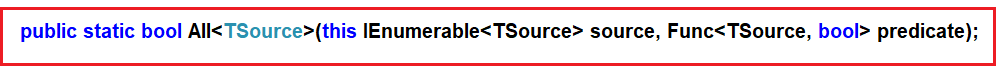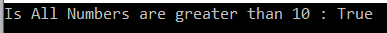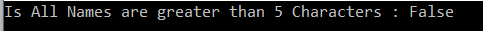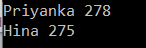# Linq All Operator in C#

## Linq All Operator in C# with Examples

In this article, I am going to discuss the Linq All Operator in C# with examples. Please read our previous article before proceeding to this article where we discussed the basics of Quantifiers Operators. As part of this article, we are going to discuss the following pointers related to Linq All Operator.

1. What is Linq All Operator?
2. Multiple examples using both Method and Query syntax.
##### What is Linq All Operator in C#?

The Linq All Operator in C# is used to check whether all the elements of a data source satisfy a given condition or not. If all the elements satisfy the condition, then it returns true else return false. There is no overloaded version is available for the All method. The definition is given below.As you can see from the above image, the Linq ALL extension method takes one predicate as a parameter.

##### Example1:

The following example returns true as all the elements are greater than 10 in the integer array.

```using System;
using System.Linq;
namespace LINQDemo
{
class Program
{
static void Main(string[] args)
{
int[] IntArray = { 11, 22, 33, 44, 55 };
var Result = IntArray.All(x => x > 10);
Console.WriteLine("Is All Numbers are greater than 10 : " + Result);
}
}
}```

Output:##### Example2:

The following example returns true as all the names are not greater than 5 characters.

```using System;
using System.Linq;
namespace LINQDemo
{
class Program
{
static void Main(string[] args)
{
string[] stringArray = { "James", "Sachin", "Sourav", "Pam", "Sara" };
var Result = stringArray.All(name => name.Length > 5);
Console.WriteLine("Is All Names are greater than 5 Characters : " + Result);
}
}
}
```

Output:##### Working with Complex Type:

We are going to work with the following Student and Subject class. So, create a class file with the name Student.cs and then copy and paste the following code.

```using System.Collections.Generic;
namespace LINQDemo
{
public class Student
{
public int ID { get; set; }
public string Name { get; set; }
public int TotalMarks { get; set; }
public List<Subject> Subjects { get; set; }

public static List<Student> GetAllStudnets()
{
List<Student> listStudents = new List<Student>()
{
new Student{ID= 101,Name = "Preety", TotalMarks = 265,
Subjects = new List<Subject>()
{
new Subject(){SubjectName = "Math", Marks = 80},
new Subject(){SubjectName = "Science", Marks = 90},
new Subject(){SubjectName = "English", Marks = 95}
}},
new Student{ID= 102,Name = "Priyanka", TotalMarks = 278,
Subjects = new List<Subject>()
{
new Subject(){SubjectName = "Math", Marks = 90},
new Subject(){SubjectName = "Science", Marks = 95},
new Subject(){SubjectName = "English", Marks = 93}
}},
new Student{ID= 103,Name = "James", TotalMarks = 240,
Subjects = new List<Subject>()
{
new Subject(){SubjectName = "Math", Marks = 70},
new Subject(){SubjectName = "Science", Marks = 80},
new Subject(){SubjectName = "English", Marks = 90}
}},
new Student{ID= 104,Name = "Hina", TotalMarks = 275,
Subjects = new List<Subject>()
{
new Subject(){SubjectName = "Math", Marks = 90},
new Subject(){SubjectName = "Science", Marks = 90},
new Subject(){SubjectName = "English", Marks = 95}
}},
new Student{ID= 105,Name = "Anurag", TotalMarks = 255,
Subjects = new List<Subject>()
{
new Subject(){SubjectName = "Math", Marks = 80},
new Subject(){SubjectName = "Science", Marks = 90},
new Subject(){SubjectName = "English", Marks = 85}
}
},
};

return listStudents;
}
}

public class Subject
{
public string SubjectName { get; set; }
public int Marks { get; set; }
}
}
```
##### Example3:

Check whether all the students having total marks greater than 250. As you can see the student James total mark is 240 which is less than 250. So here it will give you the output as false.

```using System;
using System.Linq;
namespace LINQDemo
{
class Program
{
static void Main(string[] args)
{
//Using Method Syntax
bool MSResult = Student.GetAllStudnets().All(std => std.TotalMarks > 250);

//Using Query Syntax
bool QSResult = (from std in Student.GetAllStudnets()
select std).All(std => std.TotalMarks > 250);

Console.WriteLine(MSResult);
}
}
}
```

Output: False

##### Example4:

Now we need to fetch all the student details whose mark on each subject is greater than 80.

```using System;
using System.Linq;
namespace LINQDemo
{
class Program
{
static void Main(string[] args)
{
//Using Method Syntax
var MSResult = Student.GetAllStudnets()
.Where(std => std.Subjects.All(x => x.Marks > 80)).ToList();

//Using Query Syntax
var QSResult = (from std in Student.GetAllStudnets()
where std.Subjects.All(x => x.Marks > 80)
select std).ToList();

foreach (var item in QSResult)
{
Console.WriteLine(item.Name + " " + item.TotalMarks);
}